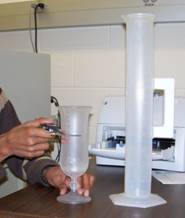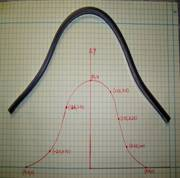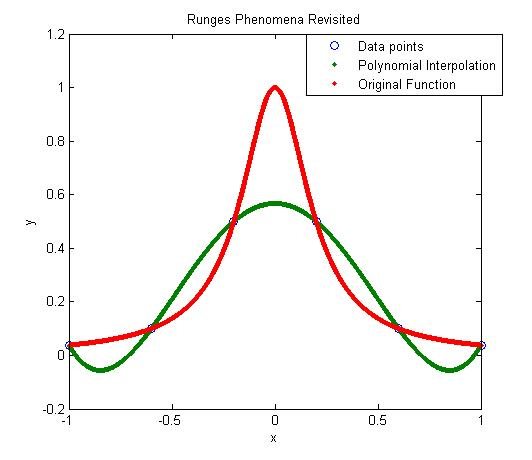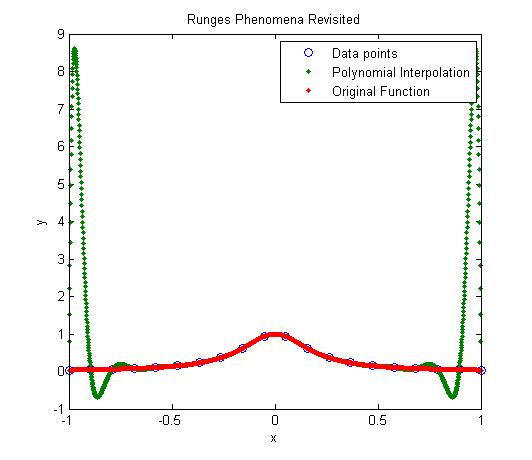## Do we have to setup all 3n equations for the n quadratic splines for (n+1) data points?

QUESTION ASKED BY STUDENT: For linear splines, we still find each line individually. In quadratic, we are forced to find every equation at once, correct? Which is where the matrix comes from?

ANSWER: That sounds right, but one can find quadratic equations individually also .
How?
If the first spline is linear, then that spline can be found by the two points it goes through.
Then the second spline goes thru two points, and that gives two equations.  The slope of the second spline is same as the slope of first spline at the common interior point, and the first spline is known. This gives the third eqn.  This process can be repeated for each following spline.

____________________________________

This post is brought to you by Holistic Numerical Methods: Numerical Methods for the STEM undergraduate at http://nm.mathforcollege.com, the textbook on Numerical Methods with Applications available from the lulu storefront, the textbook on Introduction to Programming Concepts Using MATLAB, and the YouTube video lectures available at http://nm.mathforcollege.com/videos.  Subscribe to the blog via a reader or email to stay updated with this blog. Let the information follow you.

## Experiment for spline interpolation and integration

Background:

The motivation behind the experiment is to understand spline interpolation and numerical integration by finding the volume of water that can be held by a champagne glass.

What does the student do in the lab:

The student chooses one of the odd-shaped champagne glasses (Figure 1). The student measures the outer radius of the champagne glass at different known locations along the height. The student measures the thickness of the glass, so that he/she will be able to find the inner radius of the champagne glass at the locations he/she measured the outer radius. The student pours water to the brim in the champagne glass and checks how much volume the champagne glass holds.Exercises assigned to the students:
Use MATLAB to solve problems. Use comments, display commands and fprintf statements, sensible variable names and units to explain your work. Staple all the work in the following sequence. Use USCS system of units throughout.

1. Attach the data sheet on which you collected the data in class.
2. Find the spline interpolant that curve fits the radius vs height data.
3. Show the individual points and the spline interpolant of radius vs height on a single plot.
4. Find how much volume of water the champagne glass would hold.
5. Compare the above result from problem#4 to the actual volume.
6. In 100-200 words, type out your conclusions using a word processor. Any formulas should be shown using an equation editor. Any sketches need to be drawn using a drawing software such as Word Drawing. Any plots can be imported from MATLAB.

What materials do you need; where do I buy it; how much do the materials costs?

1. Champagne Glasses: These glasses, called the Hurricane Plastic Glasses, are available at www.poolsidepineapple.com, part nos. HUR-105, HUR-106, YAR-114. We used glasses made of plastic to avoid breakage. http:/www.poolsidepineapple.com/cart_pages/shopping%20page%20tropical.htm. You can try other places to buy the champagne glasses. About \$40 or so for about six pieces including S&H. Better yet, go to a cruise and get souvenir glasses. Whenever you do the experiment, you will remember the good times.
2. Graduated Cylinder: The graduated cylinder is available at http://scientificsonline.com/, part number 3036286. The cost of the cylinder is \$20+S&H.
3. Vernier Caliper: The caliper is available at http://mcmaster.com part number 20265A49. The cost of the vernier caliper is \$60+S&H.
4. Scale: Need to buy a thin scale for this. Any art-supplies store for \$2 or so.

_____________________________________________________

This post is brought to you by Holistic Numerical Methods: Numerical Methods for the STEM undergraduate at http://nm.mathforcollege.com

## Length of a curve experiment

In a previous post, I mentioned that I have incorporated experiments in my Numerical Methods course. Here I will discuss the second experiment.In this experiment, we find the length of two curves generated from the same points – one curve is a polynomial interpolant and another one is a spline interpolant.

Motivation behind the experiment: In 1901, Runge conducted a numerical experiment to show that higher order interpolation is a bad idea. It was shown that as you use higher order interpolants to approximate f(x)=1/(1+25x2) in [-1,1], the differences between the original function and the interpolants becomes worse. This concept also becomes the basis why we use splines rather than polynomial interpolation to find smooth paths to travel through several discrete points.

What do students do in the lab: A flexible curve (see Figure) of length 12″ made of lead-core construction with graduations in both millimeters and inches is provided. The student needs to draw a curve similar in shape to the Runge’s curve on the provided graphing paper as shown. It just needs to be similar in shape – the student can make the x-domain shorter and the maximum y-value larger or vice-versa. The student just needs to make sure that there is a one-to-one correspondence of values.

Assigned Exercises: Use MATLAB to solve problems (3 thru 6). Use comments, display commands and fprintf statements, sensible variable names and units to explain your work. Staple all the work in the following sequence.

1. Signed typed affidavit sheet.
2. Attach the plot you drew in the class. Choose several points (at least nine – do not need to be symmetric) along the curve, including the end points. Write out the co-ordinates on the graphing paper curve as shown in the figure.
3. Find the polynomial interpolant that curve fits the data. Output the coefficients of the polynomial.
4. Find the cubic spline interpolant that curve fits the data. Just show the work in the mfile.
5. Illustrate and show the individual points, polynomial and cubic spline interpolants on a single plot.
6. Find the length of the two interpolants – the polynomial and the spline interpolant. Calculate the relative difference between the length of each interpolant and the actual length of the flexible curve.
7. In 100-200 words, type out your conclusions using a word processor. Any formulas should be shown using an equation editor. Any sketches need to be drawn using a drawing software such as Word Drawing. Any plots can be imported from MATLAB.

Where to buy the items for the experiment:

1. Flexible curves – I bought these via internet at Art City. The brand name is Alvin Tru-Flex Graduated Flexible Curves. Prices range from \$5 to \$12. Shipping and handling is extra – approximately \$6 plus 6% of the price. You may want to buy several 12″ and 16″ flexible curves. I had to send a query to the vendor when I did not receive them within a couple of weeks. Alternatively, call your local Art Store and see if they have them.
2. Engineering Graph Paper – Staples or Office Depot. Costs about \$12 for a pack for 100-sheet pad.
3. Pencil – Anywhere – My favorite store is the 24-hour Wal-Mart Superstore. \$1 for a dozen.
4. Scale – Anywhere – My favorite store is the 24-hour Wal-Mart Superstore. \$1 per unit.

This post is brought to you by Holistic Numerical Methods: Numerical Methods for the STEM undergraduate at http://nm.mathforcollege.com

## High order interpolation is a bad idea?

One would intuitively assume that if one was given 100 data points of data, it would be most accurate to interpolate the 100 data points to a 99th order polynomial. More the merrier: is that not the motto. But I am sorry to burst your bubble – high order interpolation is generally a bad idea.

The classic example (dating as back as 1901 before there were calculators) to show that higher order interpolation idea is a bad idea comes from the famous German mathematician Carl Runge.

He took a simple and smooth function, f(x) = 1/(1+25x^2) in the domain [-1,1]. He chose equidisant x-data points on the function f(x) in the domain xε[-1,1]. Then he used those data points to draw a polynomial interpolant.

For example, if I chose 6 data points equidistantly spaced on [-1,1], those are (-1, 0.038462), (-0.6, 0.1), (-0.2,0.5), (0.2,0.5), (0.6,0.1), and (1,0.038462). I can interpolate these 6 data points by a 5th order polynomial. In Figure 1, I am then plotting the fifth order polynomial and the original function. You can observe that there is a large difference between the original function and the polynomial interpolant. The polynomial does go through the six points, but at many other points it does not even come close to the original function. Just look at x= 0.85, the value of the function is 0.052459, but the fifth order polynomial gives you a value of -0.055762, a whopping 206.30 % error; also note the opposite sign.Figure 1. 5th order polynomial interpolation with six equidistant points.

Maybe I am not taking enough data points. Six points may be too small a number to approximate the function. Ok! Let’s get crazy. How about 20 points? That will give us a 19th order polynomial. I will choose 20 points equidistantly in [-1,1].Figure 2. 19th order polynomial interpolation with twenty equidistant points

It is not any better though it did do a better job of approximating the function except near the ends. At the ends, it is worse than before. At our chosen point, x= 0.85, the value of the function is 0.052459, while we get -0.62944 from the 19th order polynomial, and that is a big whopper error of 1299.9 %.

So are you convinced that higher order interpolation is a bad idea? Try an example by yourself: step function y=-1, -1<x<0 and y=+1, 0<x<1.

What is the alternative to higher order interpolation? Is it lower order interpolation, but does that not use data only from less points than are given. How can we use lower order polynomials and at the same time use information from all the given data points. The answer to that question is SPLINE interpolation.

This post is brought to you by Holistic Numerical Methods: Numerical Methods for the STEM undergraduate at http://nm.mathforcollege.com